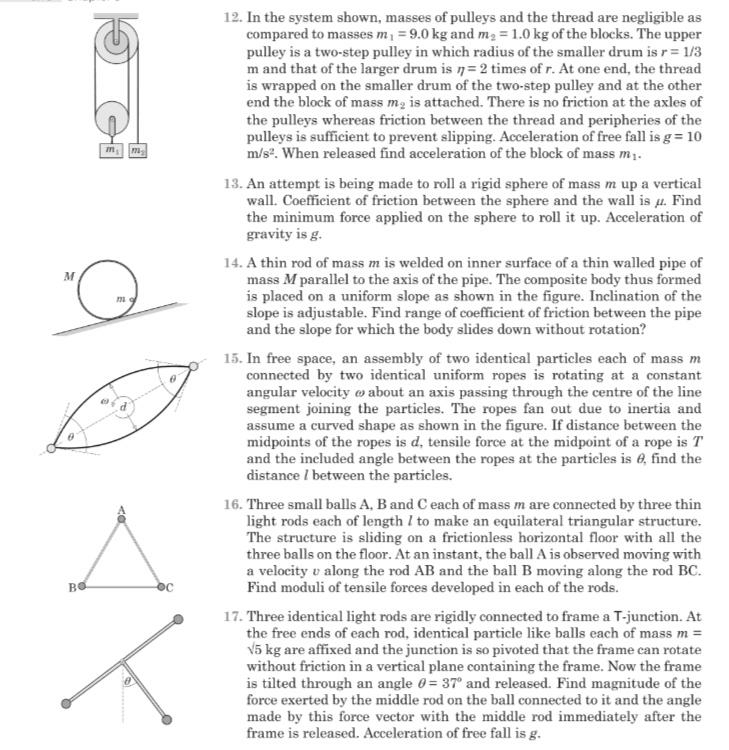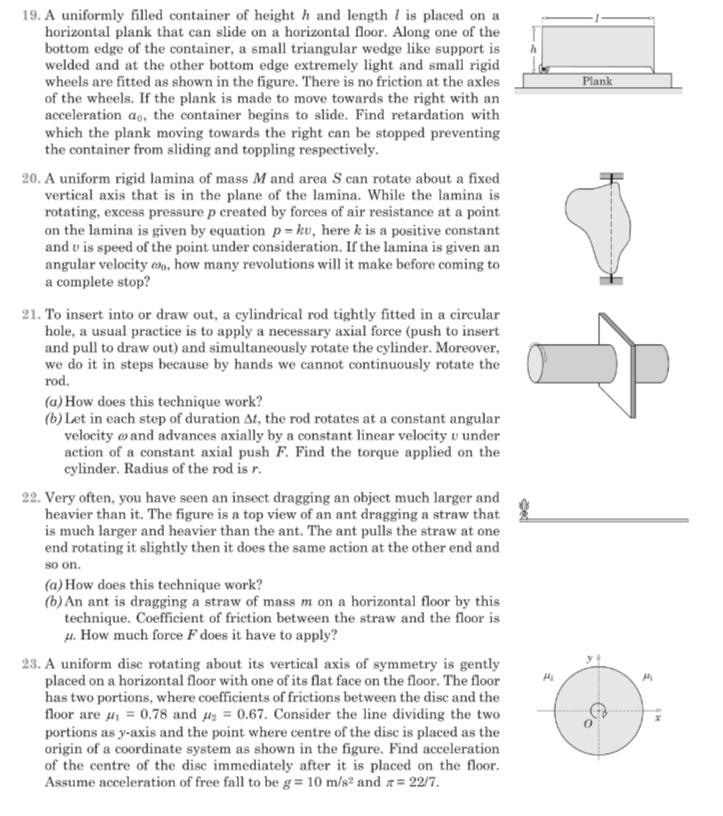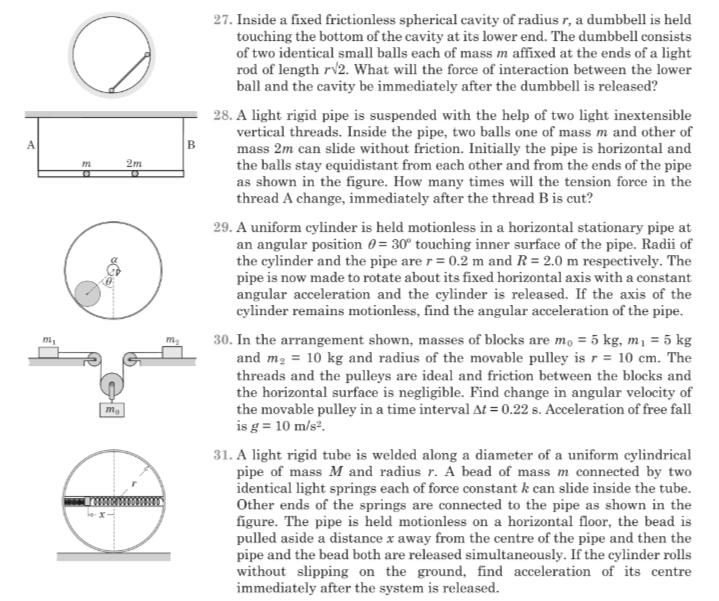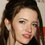# Mechanics Doubt HELP!

Here are some problems.if you have solved any one or more please post it's solutions.The question number $20, 28,31$ are resolved.If anyone want to see my attempt for a particular problem, they can ask me I will happily show the attempt.
Thanks in advance.Note by Talulah Riley
8 months, 3 weeks ago

This discussion board is a place to discuss our Daily Challenges and the math and science related to those challenges. Explanations are more than just a solution — they should explain the steps and thinking strategies that you used to obtain the solution. Comments should further the discussion of math and science.

When posting on Brilliant:

• Use the emojis to react to an explanation, whether you're congratulating a job well done , or just really confused .
• Ask specific questions about the challenge or the steps in somebody's explanation. Well-posed questions can add a lot to the discussion, but posting "I don't understand!" doesn't help anyone.
• Try to contribute something new to the discussion, whether it is an extension, generalization or other idea related to the challenge.
• Stay on topic — we're all here to learn more about math and science, not to hear about your favorite get-rich-quick scheme or current world events.

MarkdownAppears as
*italics* or _italics_ italics
**bold** or __bold__ bold
- bulleted- list
• bulleted
• list
1. numbered2. list
1. numbered
2. list
Note: you must add a full line of space before and after lists for them to show up correctly
paragraph 1paragraph 2

paragraph 1

paragraph 2

[example link](https://brilliant.org)example link
> This is a quote
This is a quote
    # I indented these lines
# 4 spaces, and now they show
# up as a code block.

print "hello world"
# I indented these lines
# 4 spaces, and now they show
# up as a code block.

print "hello world"
MathAppears as
Remember to wrap math in $$ ... $$ or $ ... $ to ensure proper formatting.
2 \times 3 $2 \times 3$
2^{34} $2^{34}$
a_{i-1} $a_{i-1}$
\frac{2}{3} $\frac{2}{3}$
\sqrt{2} $\sqrt{2}$
\sum_{i=1}^3 $\sum_{i=1}^3$
\sin \theta $\sin \theta$
\boxed{123} $\boxed{123}$

## Comments

Sort by:

Top Newest

Is the answer to problem 27 $1.5 mg$?

- 8 months, 3 weeks ago

Log in to reply

@Karan Chatrath $\Huge YES$
I am even not able to understand what the question wants to say?
Can you help me to understand what it is saying?

- 8 months, 3 weeks ago

Log in to reply

I will post a note later.

- 8 months, 3 weeks ago

Log in to reply

@Steven Chase @Karan Chatrath Please sir can you help me.. Thanks in advance.

- 8 months, 3 weeks ago

Log in to reply

I'm getting approximately $0.35$ for Problem 23. Is that the correct answer?

- 8 months, 3 weeks ago

Log in to reply

@Steven Chase $\Huge YES$

- 8 months, 3 weeks ago

Log in to reply

My answer for problem 13 is:

$F_{min} = \frac{mg}{\sqrt{49 \mu^2 + 1}}$

Is it correct?

- 8 months, 3 weeks ago

Log in to reply

@Karan Chatrath No. In the answer the coefficient of $\mu^{2}$ is $1$ instead of $49$.

- 8 months, 2 weeks ago

Log in to reply

I am managing to get that answer but somehow that result is not making sense to me.

- 8 months, 2 weeks ago

Log in to reply

Okay, I have now understood the problem. What I did is also not wrong. I analysed a slightly different case.

- 8 months, 2 weeks ago

Log in to reply

@Karan Chatrath Yeah Nice. Can you show the solution?
OR would you like to see my attempt?

- 8 months, 2 weeks ago

Log in to reply

Problem 13 solution:

Consider the force $F$ to have a horizontal and vertical component $F_x$ and $F_y$ respectively. Drawing a free body diagram will give you the following equations:

$N = F_x$ $F_y - mg - f = ma$ $fR = \frac{2mR^2}{5} \alpha$

Now for the sphere to just start rolling upwards, it must just start linearly accelerating. In the limiting case, this happens when $a=0$ and $f = \mu N$. Note that $a$ and $\alpha$ are unrelated. I was initially treating this as a pure rolling problem, but the problem does not specify that. This is a case of 'not pure rolling'. So plugging this understanding into the second equation above gives:

$F_y = \mu F_x + mg$

Now, the resultant force is:

$F = \sqrt{F_x^2+F_y^2}$

The force $F$ is minimum when the expression in the square root is minimum. Therefore, we have a constrained minimisation problem. We are required to minimise the following function $G$:

$G(F_x,F_y) = F_x^2+F_y^2$

Such that:

$F_y = \mu F_x + mg$

Solving this optimisation problem is something you should try yourself. The answer comes out to be:

$F_{min} = \frac{mg}{\sqrt{\mu^2 + 1}}$

- 8 months, 2 weeks ago

Log in to reply

@Karan Chatrath Thanks for the solution.
I am trying to understand it. By the way ,the last 3rd step of your solution seems me incorrect, according to dimension. Isn't it.?

- 8 months, 2 weeks ago

Log in to reply

I have used $\alpha$ for angular acceleration and $a$ for linear acceleration. Both symbols look similar.

- 8 months, 2 weeks ago

Log in to reply

@Karan Chatrath LAST 3rd step sir?

- 8 months, 2 weeks ago

Log in to reply

@Karan Chatrath g is a gravitational acceleration and F is a force.?

- 8 months, 2 weeks ago

Log in to reply

Ah, good catch! I meant to define a function $G$ of $F_x$ and $F_y$. I have modified the solution.

- 8 months, 2 weeks ago

Log in to reply

@Karan Chatrath i didn't understand how did you optimised that at the last. Please elaborate the solution. Thanks in advance.

- 8 months, 2 weeks ago

Log in to reply

Maxima and minima problem. Find the minimum value of the function:

$G = F_x^2 + F_y^2$

Such that: $F_y = \mu F_x + mg$

Write $F_y$ in terms of $F_x$ and you have a function of $F_x$ only. Do you know the next step?

- 8 months, 2 weeks ago

Log in to reply

@Karan Chatrath Yes i got it. The next step is differentiation and then just squaring.
But the most important thing is the moment of inertia is not used in this problem which seems me bit surprising.

- 8 months, 2 weeks ago

Log in to reply

I used moment of inertia when I treated this problem as a case of pure rolling. That is why I got a factor of 49 multiplied with $\mu^2$. But since it is not a pure rolling case, $a \ne R\alpha$ and therefore, the moment of inertia dependence is lost.

- 8 months, 2 weeks ago

Log in to reply

@Karan Chatrath Exactly. It means friction is acting in the ball. Thanks By the way try the 14th question.

- 8 months, 2 weeks ago

Log in to reply

@Karan Chatrath i didn't understand the solution of this problem
Can you post a very explanatory solution.
After reading word rolling, my brain stops working . :)

- 8 months, 2 weeks ago

Log in to reply

×

Problem Loading...

Note Loading...

Set Loading...